# Math in Focus Grade 3 Chapter 14 Practice 5 Answer Key Adding and Subtracting Like Fractions

Practice the problems of Math in Focus Grade 3 Workbook Answer Key Chapter 14 Practice 5 Adding and Subtracting Like Fractions to score better marks in the exam.

## Math in Focus Grade 3 Chapter 14 Practice 5 Answer Key Adding and Subtracting Like Fractions

Complete the model. Add the fractions.

Example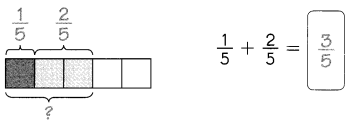Question 1.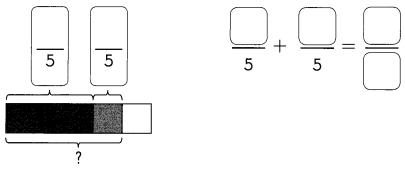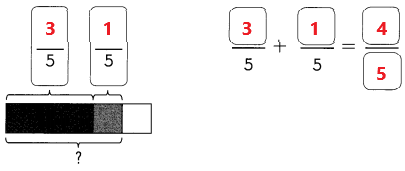Explanation:
In the above image we can observe 3/5 part is shaded with black color and 1/5 part is shaded with gray color. Add the fraction 3/5 with 1/5 the sum is 4/5.

Question 2.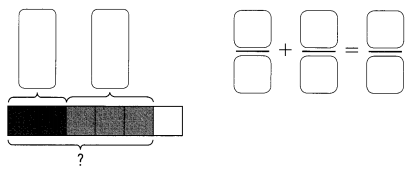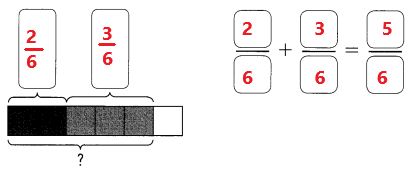Explanation:
In the above image we can observe 2/6 part is shaded with black color and 3/6 part is shaded with gray color. Add the fraction 2/6 with 3/6 the sum is 5/6.

Question 3.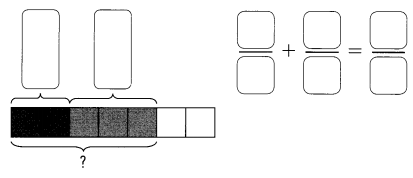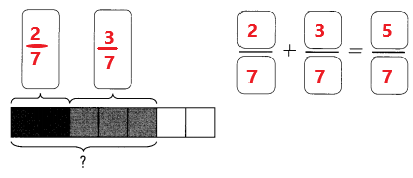Explanation:
In the above image we can observe 2/7 part is shaded with black color and 3/7 part is shaded with gray color. Add the fractions 2/7 with 3/7 the sum is 5/7.

Complete.

Question 4.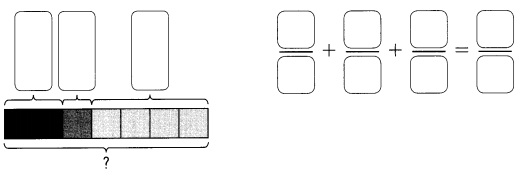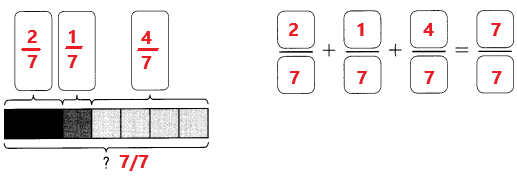Explanation:
In the above image we can observe 2/7 part is shaded with black color and 1/7 part is shaded with gray color and 4/7 part is shaded with white color. Add the fractions 2/7 and 1/7 with 4/7 the sum is 7/7.

Question 5.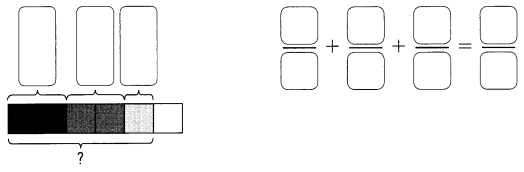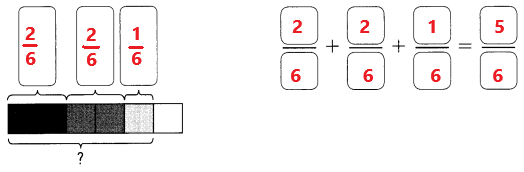Explanation:
In the above image we can observe 2/6 part is shaded with black color and 2/6 part is shaded with gray color and 1/6 part is shaded with white color. Add the fractions 2/6 and 2/6 with 1/6 the sum is 5/6.

Question 6.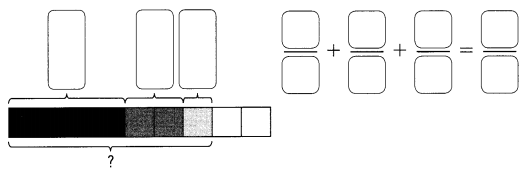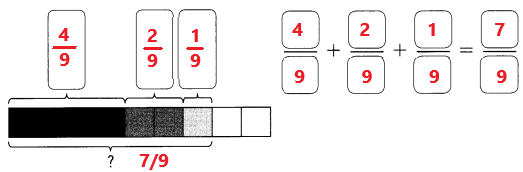Explanation:
In the above image we can observe 4/9 part is shaded with black color and 2/9 part is shaded with gray color and 1/9 part is shaded with white color. Add the fractions 4/9 and 2/9 with 1/9 the sum is 7/9.

Question 7.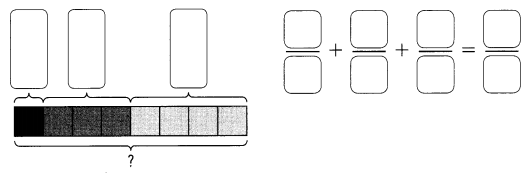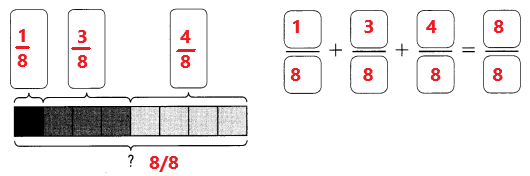Explanation:
In the above image we can observe 1/8 part is shaded with black color and 3/8 part is shaded with gray color and 4/8 part is shaded with white color. Add the fractions 1/8 and 3/8 with 4/8 the sum is 8/8.

Complete the model. Subtract the fractions.

Example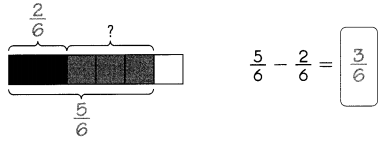Question 8.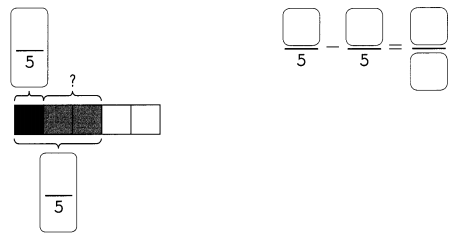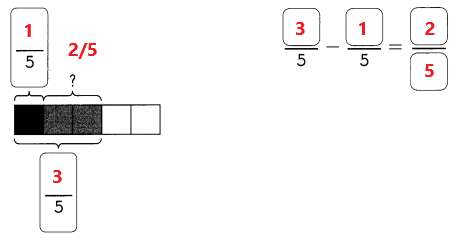Explanation:
In the above image we can observe 1/5 part is shaded with black color and 3/5 part is shaded with both black and gray colors. We have to find out gray color shaded part. Subtract 1/5 from 3/5 the difference is 2/5. 2/5 part is shaded with gray color.

Question 9.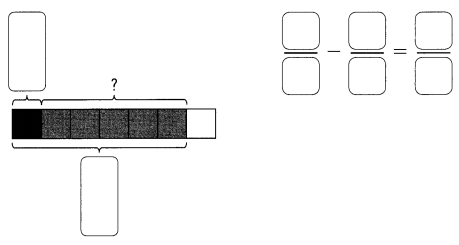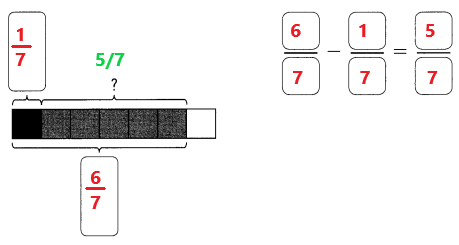Explanation:
In the above image we can observe 1/7 part is shaded with black color and 6/7 part is shaded with both black and gray colors. We have to find out gray color shaded part. Subtract 1/7 from 6/7 the difference is 5/7. 5/7 part is shaded with gray color.

Complete.

Question 10.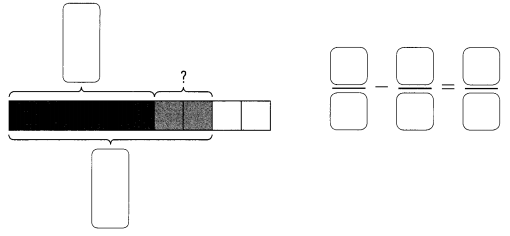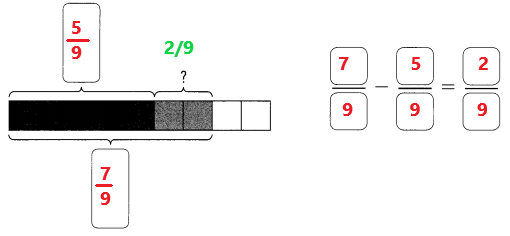Explanation:
In the above image we can observe 5/9 part is shaded with black color and 7/9 part is shaded with both black and gray colors. We have to find out gray color shaded part. Subtract 5/9 from 7/9 the difference is 2/9. 2/9 part is shaded with gray color.

Question 11.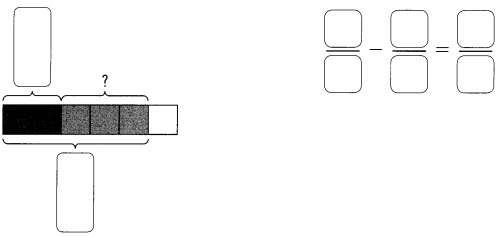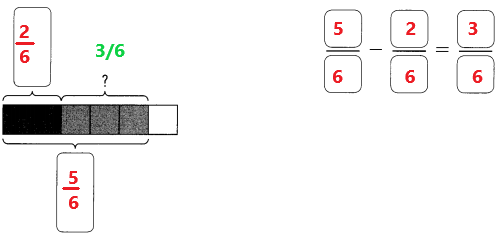Explanation:
In the above image we can observe 2/6 part is shaded with black color and 5/6 part is shaded with both black and gray colors. We have to find out gray color shaded part. Subtract 2/6 from 5/6 the difference is 3/6. 3/6 part is shaded with gray color.

Question 12.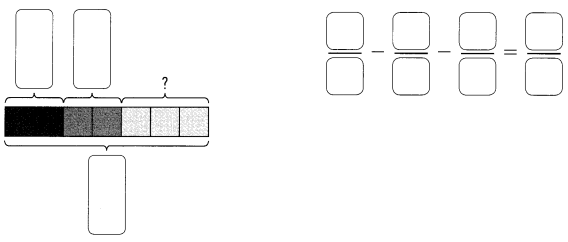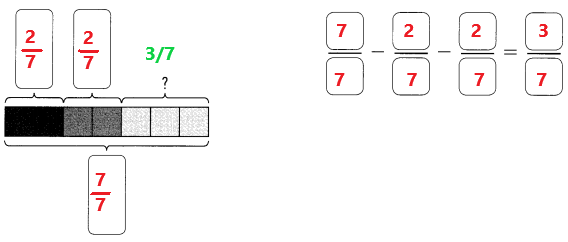Explanation:
In the above image we can observe 2/7 part is shaded with black color, 2/7 part is shaded with gray color and 7/7 part is shaded with black, gray and white colors. We have to find out white color shaded part. Subtract 2/7 and 2/7 from7/7 the difference is 3/7. 3/7 part is shaded with white color.

Question 13.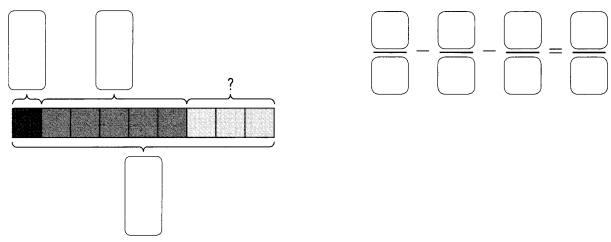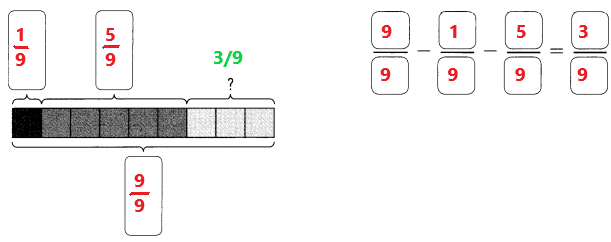Explanation:
In the above image we can observe 1/9 part is shaded with black color, 5/9 part is shaded with gray color and 9/9 part is shaded with black, gray and white colors. We have to find out white color shaded part. Subtract 1/9 and 5/9 from 9/9 the difference is 3/9. 3/9 part is shaded with white color.

Question 14.
$$\frac{1}{4}+\frac{2}{4}$$ = _______________
1/4 + 2/4 = 3/4
Explanation:
Perform addition operation on above two fractions. Add 1/4 with 2/4 the sum is 3/4.

Question 15.
$$\frac{2}{6}+\frac{3}{6}$$ = _______________
2/6 + 3/6 = 5/6
Explanation:
Perform addition operation on above two fractions. Add 2/6 with 3/6 the sum is 5/6.

Question 16.
$$\frac{2}{5}+\frac{2}{5}$$ = _______________
2/5 + 2/5 = 4/5
Explanation:
Perform addition operation on above two fractions. Add 2/5 with 2/5 the sum is 4/5.

Question 17.
$$\frac{3}{7}+\frac{1}{7}$$ = _______________
3/7 + 1/7 = 4/7
Explanation:
Perform addition operation on above two fractions. Add 3/7 with 1/7 the sum is 4/7.

Question 18.
$$\frac{2}{9}+\frac{5}{9}$$ = _______________
2/9 + 5/9 = 7/9
Explanation:
Perform addition operation on above two fractions. Add 2/9 with 5/9 the sum is 7/9.

Question 19.
$$\frac{5}{11}+\frac{6}{11}$$ = _______________
5/11 + 6/11 = 11/11 = 1
Explanation:
Perform addition operation on above two fractions. Add 5/11 with 6/11 the sum is 11/11. The simplified form of 11/11 is 1.

Question 20.
$$\frac{1}{8}+\frac{4}{8}$$ = _______________
1/8 + 4/8 = 5/8
Explanation:
Perform addition operation on above two fractions. Add 1/8 with 4/8 the sum is 5/8.

Question 21.
$$\frac{1}{4}+\frac{3}{4}$$ = _______________
1/4 + 3/4 = 4/4 = 1
Explanation:
Perform addition operation on above two fractions. Add 1/4 with 3/4 the sum is 4/4. The fraction 4/4 in simplified form is 1.

Question 22.
$$\frac{1}{6}+\frac{5}{6}$$ = _______________
1/6 + 5/6 = 6/6 = 1
Explanation:
Perform addition operation on above two fractions. Add 1/6 with 5/6 the sum is 6/6. The fraction 6/6 in simplified form is 1.

Question 23.
$$\frac{3}{9}+\frac{1}{9}$$ = _______________
3/9 + 1/9 = 4/9
Explanation:
Perform addition operation on above two fractions. Add 3/9 with 1/9 the sum is 4/9.

Question 24.
$$\frac{2}{10}+\frac{3}{10}+\frac{4}{10}$$ = _______________
2/10 + 3/10 + 4/10 = 9/10
Explanation:
Perform addition operation on above three fractions. Add 2/10 and 3/10 with 4/10 the sum is 9/10.

Question 25.
$$\frac{5}{12}+\frac{4}{12}+\frac{2}{12}$$ = _______________
5/12 + 4/12 + 2/12 = 11/12
Explanation:
Perform addition operation on above three fractions. Add 5/12 and 4/12 with 2/12 the sum is 11/12.

Question 26.
$$\frac{2}{6}+\frac{1}{6}+\frac{2}{6}$$ = _______________
2/6 + 1/6 + 2/6 = 5/6
Explanation:
Perform addition operation on above three fractions. Add 2/6 and 1/6 with 2/6 the sum is 5/6.

Question 27.
$$\frac{5}{8}+\frac{1}{8}+\frac{2}{8}$$ = _______________
5/8 + 1/8 + 2/8 = 8/8 = 1
Explanation:
Perform addition operation on above three fractions. Add 5/8 and 1/8 with 2/8 the sum is 8/8. The fraction 8/8 in simplified form is 1.

Question 28.
$$\frac{3}{9}+\frac{1}{9}+\frac{4}{9}$$ = _______________
3/9 + 1/9 + 4/9 = 8/9
Explanation:
Perform addition operation on above three fractions. Add 3/9 and 1/9 with 4/9 the sum is 8/9.

Question 29.
$$\frac{1}{7}+\frac{4}{7}+\frac{1}{7}$$ = _______________
1/7 + 4/7 + 1/7 = 6/7
Explanation:
Perform addition operation on above three fractions. Add 1/7 and 4/7 with 1/7 the sum is 6/7.

Question 30.
$$\frac{1}{4}+\frac{1}{4}+\frac{1}{4}$$ = _______________
1/4 + 1/4 + 1/4 = 3/4
Explanation:
Perform addition operation on above three fractions. Add 1/4 and 1/4 with 1/4 the sum is 3/4.

Question 31.
$$\frac{2}{5}+\frac{1}{5}+\frac{1}{5}$$ = _______________
2/5 + 1/5 + 1/5 = 4/5
Explanation:
Perform addition operation on above three fractions. Add 2/5 and 1/5 with 1/5 the sum is 4/5.

Subtract.

Question 32.
$$\frac{4}{5}-\frac{2}{5}$$ = _______________
4/5 – 2/5 = 2/5
Explanation:
Perform subtraction operation on above two fractions. Subtract 2/5 from 4/5 the difference is 2/5.

Question 33.
$$\frac{5}{6}-\frac{4}{6}$$ = _______________
5/6 – 4/6 = 1/6
Explanation:
Perform subtraction operation on above two fractions. Subtract 4/6 from 5/6 the difference is 1/6.

Question 34.
$$\frac{8}{9}-\frac{4}{9}$$ = _______________
8/9 – 4/9 = 4/9
Explanation:
Perform subtraction operation on above two fractions. Subtract 4/9 from 8/9 the difference is 4/9.

Question 35.
$$\frac{9}{11}-\frac{3}{11}$$ = _______________
9/11 – 3/11 = 6/11
Explanation:
Perform subtraction operation on above two fractions. Subtract 3/11 from 9/11 the difference is 6/11.

Question 36.
$$\frac{6}{7}-\frac{3}{7}$$ = _______________
6/7 – 3/7 = 3/7
Explanation:
Perform subtraction operation on above two fractions. Subtract 3/7 from 6/7 the difference is 3/7.

Question 37.
$$\frac{11}{12}-\frac{4}{12}$$ = _______________
11/12 – 4/12 = 7/12
Explanation:
Perform subtraction operation on above two fractions. Subtract 4/12 from 11/12 the difference is 7/12.

Question 38.
$$\frac{5}{6}-\frac{1}{6}$$ = _______________
5/6 – 1/6 = 4/6
Explanation:
Perform subtraction operation on above two fractions. Subtract 1/6 from 5/6 the difference is 4/6.

Question 39.
$$\frac{7}{8}-\frac{3}{8}$$ = _______________
7/8 – 3/8 = 4/8
Explanation:
Perform subtraction operation on above two fractions. Subtract 3/8 from 7/8 the difference is 4/8.

Question 40.
$$1-\frac{7}{9}$$ = _______________
1 – 7/9 = (9 – 7)/9 = 2/9
Explanation:
Perform subtraction operation on above two fractions. Subtract 7/9 from 1 the difference is 2/9.

Question 41.
$$1-\frac{5}{10}$$ = _______________
1 – 5/10 = (10 – 5)/10 = 5/10 = 1/2
Explanation:
Perform subtraction operation on above two fractions. Subtract 5/10 from 1 the difference is 5/10. The simplified form of the fraction 5/10 is 1/2.

Question 42.
$$\frac{8}{12}-\frac{1}{12}-\frac{2}{12}$$ = _______________
8/12 – 1/12 – 2/12 = (8 – 1 – 2)/12 = 5/12
Explanation:
Perform subtraction operation on above three fractions. Subtract 1/12 and 2/12 from 8/12 the difference is 5/12.

Question 43.
$$\frac{9}{12}-\frac{1}{12}-\frac{3}{12}$$ = _______________
9/12 – 1/12 – 3/12 = (9 – 1 – 3)/12 = 5/12
Explanation:
Perform subtraction operation on above three fractions. Subtract 1/12 and 3/12 from 9/12 the difference is 5/12.

Question 44.
$$\frac{10}{12}-\frac{2}{12}-\frac{3}{12}$$ = _______________
10/12 – 2/12 – 3/12 = (10 – 2 – 3)/12 = 5/12
Explanation:
Perform subtraction operation on above three fractions. Subtract 2/12 and 3/12 from 10/12 the difference is 5/12.

Question 45.
$$\frac{8}{11}-\frac{1}{11}-\frac{5}{11}$$ = _______________
8/11 – 1/11 – 5/11 = 2/11
Explanation:
Perform subtraction operation on above three fractions. Subtract 1/11 and 5/11 from 8/11 the difference is 2/11.

Question 46.
$$\frac{8}{9}-\frac{2}{9}-\frac{3}{9}$$ = _______________
8/9 – 2/9 – 3/9 = (8 – 2 – 3)/9 = 3/9 = 1/3
Explanation:
Perform subtraction operation on above three fractions. Subtract 2/9 and 3/9 from 8/9 the difference is 3/9. The simplified form of the fraction 3/9 is 1/3.

Question 47.
$$\frac{6}{7}-\frac{1}{7}-\frac{4}{7}$$ = _______________
6/7 – 1/7 – 4/7 = (6 – 1 -4)/7 = 1/7
Explanation:
Perform subtraction operation on above three fractions. Subtract 1/7 and 4/7 from 6/7 the difference is 1/7.

Question 48.
$$1-\frac{2}{9}-\frac{3}{9}$$ = _______________
$$1-\frac{1}{7}-\frac{4}{7}$$ = _______________